#### The Data Science Lab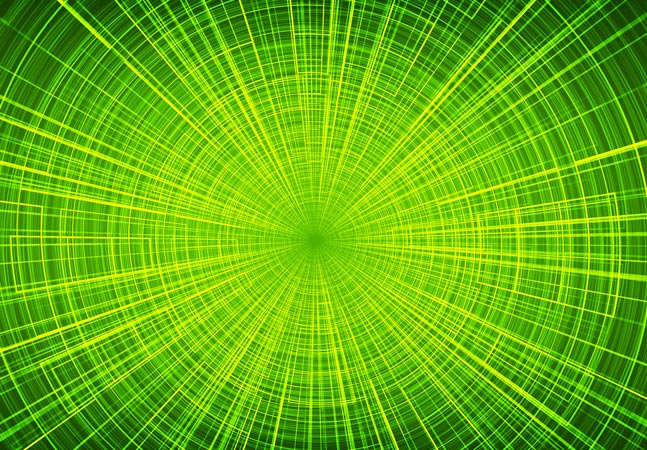### How to Do Logistic Regression Using ML.NET

Microsoft Research's Dr. James McCaffrey show how to perform binary classification with logistic regression using the Microsoft ML.NET code library. The goal of binary classification is to predict a value that can be one of just two discrete possibilities, for example, predicting if a person is male or female### Getting Started with AutoML for ML.NET

Dr. James McCaffrey provides hands-on examples in introducing ML.NET, for machine learning prediction models, and AutoML, which automatically examines different ML algorithms, finds the best one, and creates a Visual Studio project with the C# code backing the best model, along with C# code that shows how to use the trained model to make a prediction.### How to Do Neural Network Glorot Initialization Using Python

Microsoft Research data scientist Dr. James McCaffrey explains what neural network Glorot initialization is and why it's the default technique for weight initialization.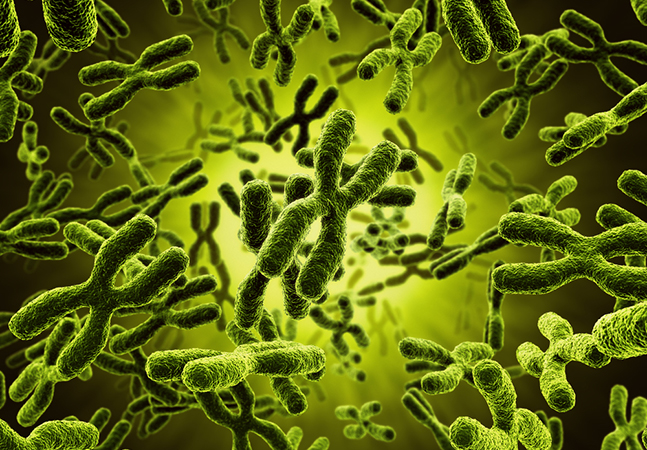### How To Code Modern Neural Networks Using Python and NumPy

Data scientist Dr. James McCaffrey begins a series on presenting and explaining the most common modern techniques used for neural networks, for which over the past couple of years there have been many small but significant changes in the default techniques used.### How to Do Thompson Sampling Using Python

Suppose you have three different Internet advertising strategies and you want to determine which of them is the best as quickly as possible. Or suppose you work for a medical company and you want to determine which of three new drugs is the most effective. Resident data scientist Dr. James McCaffrey shows how Thompson Sampling can help.### Naive Bayes Classification Using Python

Dr. James McCaffrey of Microsoft Research uses Python code samples and screenshots to explain naive Bayes classification, a machine learning technique used to predict the class of an item based on two or more categorical predictor variables, such as predicting the gender (0 = male, 1 = female) of a person based on occupation, eye color and nationality.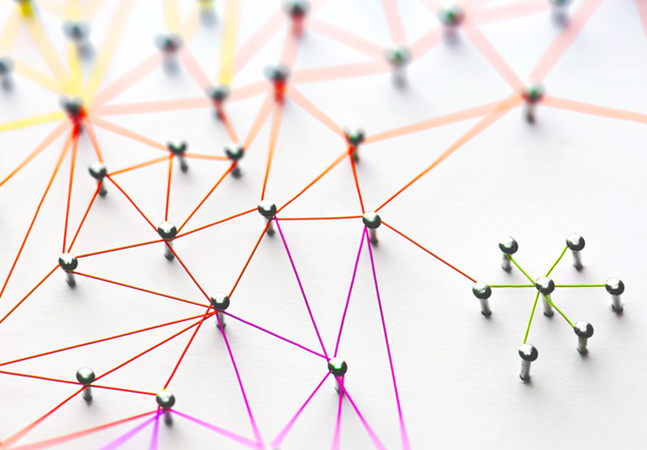### Weighted k-NN Classification Using Python

Need to predict the political party affiliation (democrat, republican, independent) of a person based on their age, annual income, gender, years of education and so on? Our resident data scientist Dr. James McCaffrey shows a technique that can help with that and much more -- with code!### Neural Anomaly Detection Using Keras

Our resident doctor of data science this month tackles anomaly detection, using code samples and screenshots to explain the process of finding rare items in a dataset, such as discovering fraudulent login events or fake news items.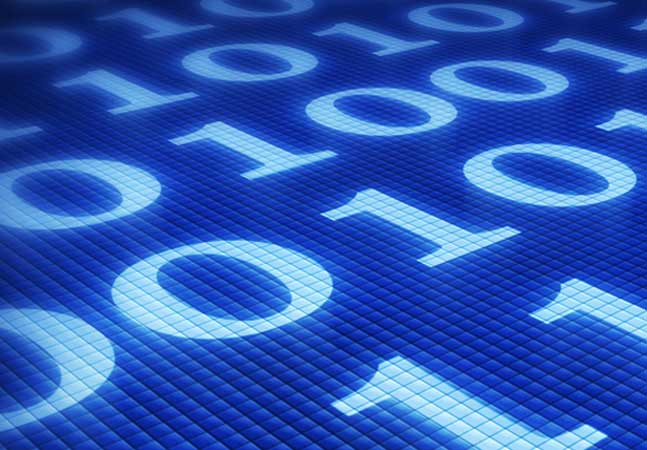### Support Vector Machines Using Accord.NET

The Data Science doctor delves into supporting vector machines, software systems that can perform binary classification such as creating a model to predict the gender of a person based on their age, annual income, height and weight.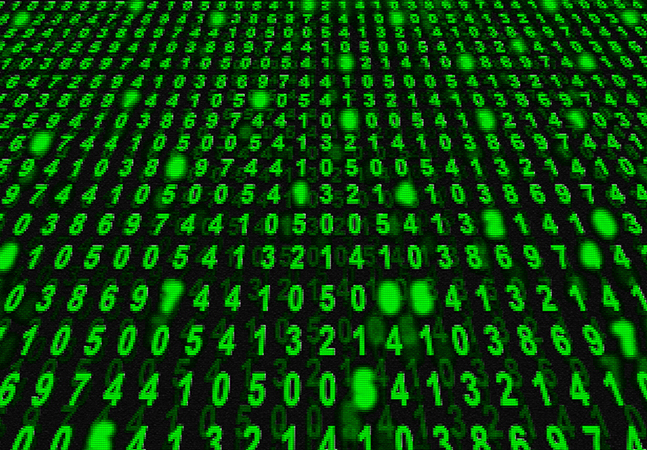### Self-Organizing Maps Using Python

Dr. James McCaffrey of Microsoft Research uses a full project code sample and screenshots to detail how to use Python to work with self-organizing maps (SOM), which let you investigate the structure of a set of data.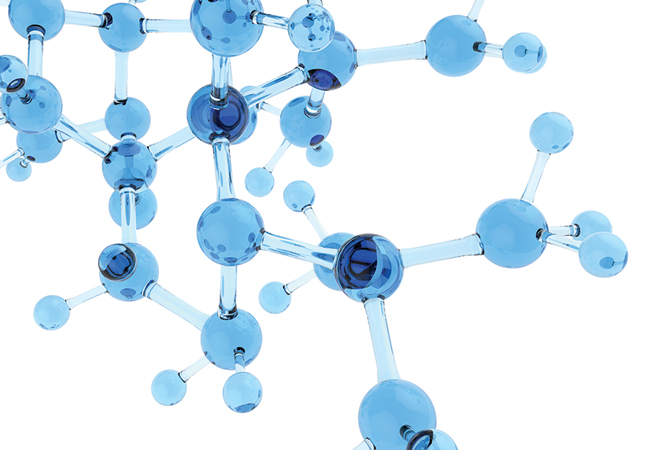### Image Classification Using Keras

Creating a custom image classification model is challenging, but the existence of neural network libraries like Keras has made it doable. Here's how, with many code samples and a full project download.### Sentiment Analysis Using Keras

Custom sentiment analysis is hard, but neural network libraries like Keras with built-in LSTM (long, short term memory) functionality have made it feasible. Step into the Data Science Lab with Dr. McCaffrey to find out how, with full code examples.### Q-Learning Using Python

The Data Science Doctor explains how to use the reinforcement learning branch of machine learning with the Q-learning approach, providing code on how to solve a maze problem for an easy-to-understand example.### How to Do Neural Binary Classification Using Keras

Our resident data scientist provides a hands-on example on how to make a prediction that can be one of just two possible values, which requires a different set of techniques than classification problems where the value to predict can be one of three or more possible values.### Neural Regression Using Keras

Data scientist Dr. James McCaffrey provides code, screenshots and step-by-step instructions on how to perform regression using a deep neural network with the Keras code library.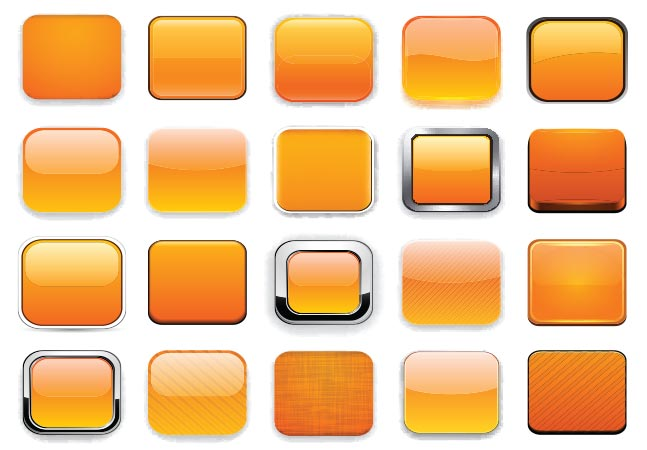### DNN Image Classification Using Keras

The Data Science Doctor provides a hands-on tutorial, complete with code samples, to explain one of the most common methods for image classification, deep neural network, used, for example, to identify a photograph of an animal as a "dog" or "cat" or "monkey."### Introduction to Keras with TensorFlow

Our data science doctor provides a hands-on neural networking tutorial to explain how to get started with the popular Keras library, a high-level wrapper over TensorFlow.### Clustering Non-Numeric Data Using Python

The data science doctor explains everything you need to know about clustering data, the process of grouping items so those in a group (cluster) are similar and items in different groups are dissimilar.### Data Clustering with K-Means Using Python

Our Data Science Lab guru explains how to implement the k-means technique for data clustering, or cluster analysis, which is the process of grouping data items so that similar items belong to the same group/cluster.### Neural Network Dropout Using Python

Go hands-on with data scientist Dr. James McCaffrey as he explains neural network dropout, a technique that can be used during training to reduce the likelihood of model overfitting.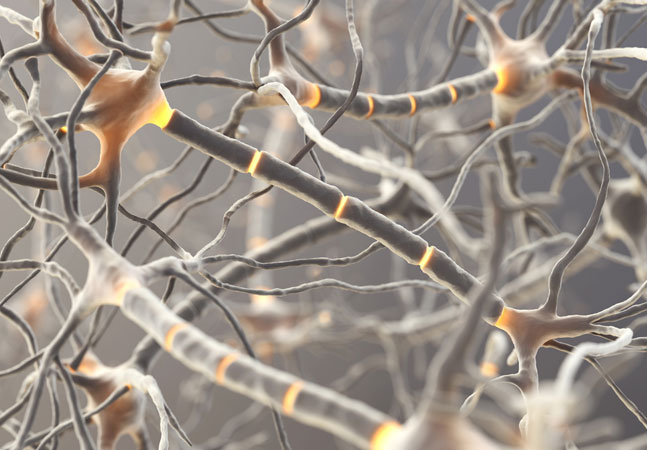### Neural Network Time Series Regression Using Python

Learn how to do time series regression using a neural network, with "rolling window" data, coded from scratch, using Python.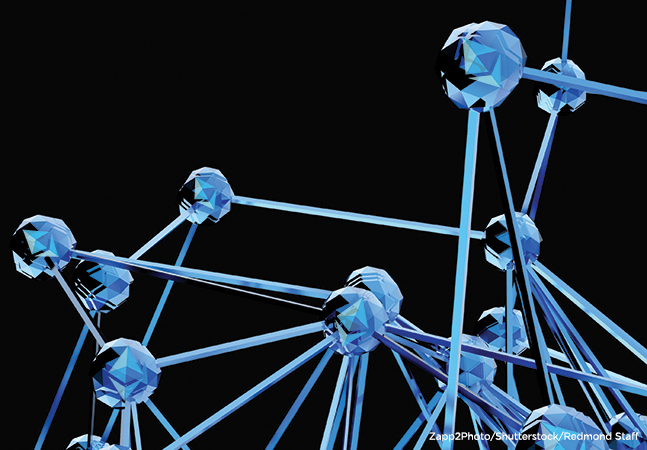### Logistic Regression Using Python

The data doctor continues his exploration of Python-based machine learning techniques, explaining binary classification using logistic regression, which he likes for its simplicity.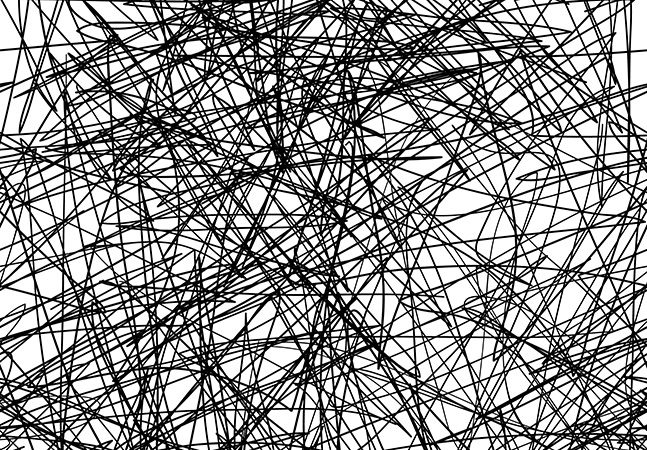### Neural Network L1 Regularization Using Python

The data science doctor continues his exploration of techniques used to reduce the likelihood of model overfitting, caused by training a neural network for too many iterations.### Neural Network Batch Training Using Python

Our resident data scientist explains how to train neural networks with two popular variations of the back-propagation technique: batch and online.### Neural Network L2 Regularization Using Python

Our data science expert continues his exploration of neural network programming, explaining how regularization addresses the problem of model overfitting, caused by network overtraining.

Upcoming Events